# Algebra II : Real Numbers

## Example Questions

### Example Question #11 : Number Theory

Using the properties of negative numbers, determine which answer is equivalent to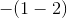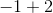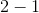Explanation:

Recall the following property of negative numbers: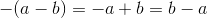It follows that: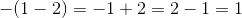### Example Question #11 : Real Numbers

Using the properties of negative numbers, determine which answer is equivalent to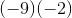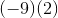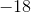Explanation:

Recall the following property of negative numbers: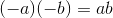It follows that: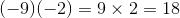### Example Question #12 : Number Theory

Solve the following using properties of fractions: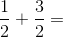Explanation: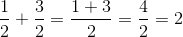### Example Question #14 : Number Theory

Solve the following using properties of fractions: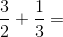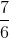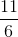Explanation: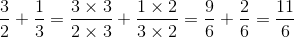### Example Question #15 : Number Theory

Solve the following using properties of fractions: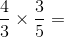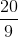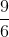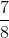Explanation: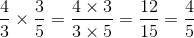### Example Question #12 : Real Numbers

Solve the following using properties of fractions: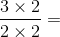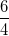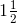Explanation: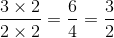Every answer choice is equivalent to this, so they are all correct.

### Example Question #17 : Number Theory

Solve the following using properties of fractions: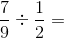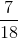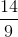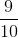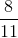Explanation:

When dividing fractions, remember to multiply the first fraction by the reciprocal of the second, as illustrated by the following identity: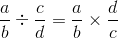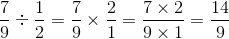### Example Question #18 : Number Theory

Find the equivalent of the following: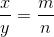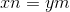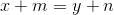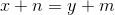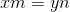Explanation:

One of the properties of fractions states that if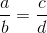, then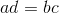.

It follows that if, then.

### Example Question #13 : Real Numbers

Which of the following is a NOT a real number?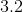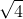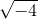Explanation:

By definition, a real number is one that is NOT imaginary. Imaginary numbers are numbers that, when squared, give a negative result.

So, the correct answer choice for the above question must be one that is, in fact, an imaginary number.

Using the above defintion, we are able to identify only one of the answer choices as a number that, when squared, gives a negative result. This is. When we take the square of, we get, ultimately, satisfying our definition of an imaginary number, and giving us the correct answer choice.

### Example Question #13 : Real Numbers

Which is NOT a real number?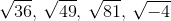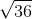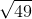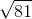Explanation:

The mathematics a real number is any integer, irrational, or rational number. The set of real numbers does not include imaginary numbers.

If we look at the possible answers we see that three out of the four are real numbers because they are integers.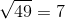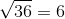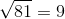7, 6, and 9 are all Real Numbers.

The answer isbecause when a negative number is under a radical sign it creates an imaginary number which is not included in the set of real numbers.

### All Algebra II Resources